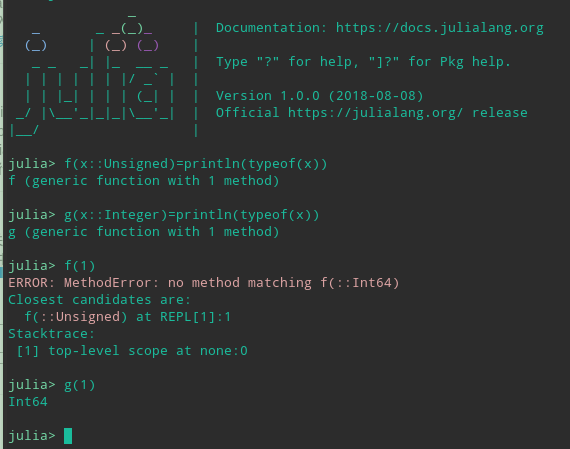# Unsigned 为什么不能作为形参类型

``````julia> mutable struct Point{T <: Real}
point::Array{T, 1}

function Point{T}(dim::Integer) where T
point = Array{T, 1}(undef, dim)
new{T}(point)
end
end

julia> function compare(p1::Point, p2::Point, dim::Unsigned)
@info "hello..."
end
compare (generic function with 1 method)

julia> compare(Point{Int64}(2), Point{Int64}(2), 2)
ERROR: MethodError: no method matching compare(::Point{Int64}, ::Point{Int64}, ::Int64)
Closest candidates are:
compare(::Point, ::Point, ::Unsigned) at REPL:2
Stacktrace:
 top-level scope at none:0

julia> function compare(p1::Point, p2::Point, dim::Integer)
@info "hello..."
end
compare (generic function with 2 methods)

julia> compare(Point{Int64}(2), Point{Int64}(2), 2)
[ Info: hello...

######################################################

julia> f(x::Unsigned)=println(typeof(x))
f (generic function with 1 method)

julia> g(x::Integer)=println(typeof(x))
g (generic function with 1 method)

julia> t(x::Signed)=println(typeof(x))
t (generic function with 1 method)

julia> f(1)
ERROR: MethodError: no method matching f(::Int64)
Closest candidates are:
f(::Unsigned) at REPL:1
Stacktrace:
 top-level scope at none:0

julia> g(1)
Int64

julia> t(1)
Int64

`````````

```

``````julia> Unsigned <: Integer
true

julia> 1 isa Unsigned
false

julia> 1 isa Integer
true
``````

``````f(x::Unsigned)=println(typeof(x))
``````